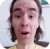FreeKB - PowerShell Get-ChildItem Count command
PowerShell - Get-ChildItem Count commandThe Get-ChildItem cmdlet with the Count option can be used to count the number of files and folders in a directory. For example, to count the number of files and folders at C:Example Directory:

``````(Get-ChildItem "C:Example Directory").Count
15``````

Recurse

This will not count files and folders below C:Example Directory. The -Recurse option can be used to count objects below C:Example Directory.

``````(Get-ChildItem "C:Example Directory" -Recurse).Count
72``````

Where clause

Let's say we want to count all of the files that have not been modified in the last 365 days.

``````\$One_year_ago = (Get-Date).AddDays(-365).ToString("yyyy-MM-dd")
\$source = "C:path	oexample.txt"
\$File_to_check = (Get-Item -Path \$source).LastWriteTime.ToString("yyyy-MM-dd")

if ((\$File_to_check) -lt (\$One_year_ago)) {"File to check less than One year ago"}``````

Measure Object

If you want to also determine the size of the directory, in bytes, KB, MB, GB, or TB, the Measure-Object option can be used. In this example, there are 72 files at and below C:Example Directory, and the size of the directory is 27,136 bytes.

``````Get-ChildItem "C:Example Directory" -Recurse | Measure-Object -property length -sum

Count    : 72
Average  :
Sum      : 67181464
Maximum  :
Minimum  :
Property : Length ``````

We can adjust the output to only show count and bytes.

``````\$y = (Get-ChildItem "C:Example Directory" -Recurse | Measure-Object -property length -sum)
echo \$y.count
echo \$y.sum

72
67181464``````

Instead of displaying 67181464 bytes, we can display the size of the files in the directory in KB (or MB, GB, TB).

``````\$y = (Get-ChildItem "C:Example Directory" -Recurse | Measure-Object -property length -sum)
echo \$y.count
echo "{0:N0}" -f (\$y.sum / 1KB) + " KB"

72
65,606 KB``````

There may be a scenario where the comma in the output is problematic. Let's drop the comma.

``````\$y = (Get-ChildItem "C:Example Directory" -Recurse | Measure-Object -property length -sum)
echo \$y.count
\$x = [math]::Round(\$y.sum) / 1024
[int]\$x

72
65606``````

We will never share your name or email with anyone. Enter your email if you would like to be notified when we respond to your comment.

Please enter aac56 in the box below so that we can be sure you are a human.

Web design by yours truely - me, myself, and I   |   jeremy.canfield@freekb.net   |